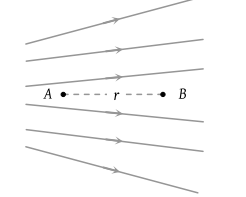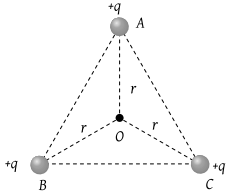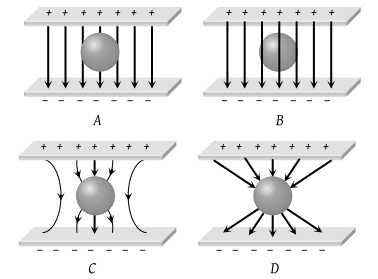Two spherical conductors B and C having equal radii and carrying equal charges in them repel each other with a force F when kept apart at some distance. A third spherical conductor having same radius as that of B but uncharged is brought in contact with B, then brought in contact with C and finally removed away from both. The new force of repulsion between B and C is

(1) F/4

(2) 3 F/4

(3) F/8

(4) 3 F/8

Concept Questions :-

Coulomb's law
High Yielding Test Series + Question Bank - NEET 2020

Difficulty Level:

Two equally charged, identical metal spheres A and B repel each other with a force 'F'. The spheres are kept fixed with a distance 'r' between them. A third identical, but uncharged sphere C is brought in contact with A and then placed at the mid-point of the line joining A and B. The magnitude of the net electric force on C is

(1) F

(2) 3F/4

(3) F/2

(4) F/4

Concept Questions :-

Electric field
High Yielding Test Series + Question Bank - NEET 2020

Difficulty Level:

An infinite number of charges, each of charge 1 μC, are placed on the x-axis with co-ordinates x = 1, 2, 4, 8, ....∞. If a charge of 1 C is kept at the origin, then what is the net force acting on 1 C charge

(1) 9000 N

(2) 12000 N

(3) 24000 N

(4) 36000 N

High Yielding Test Series + Question Bank - NEET 2020

Difficulty Level:

A charge q is placed at the centre of the line joining two equal charges Q. The system of the three charges will be in equilibrium, if q is equal to

(1) $-\frac{Q}{2}$

(2) $-\frac{Q}{4}$

(3) $+\frac{Q}{4}$

(4) $+\frac{Q}{2}$

Concept Questions :-

Coulomb's law
High Yielding Test Series + Question Bank - NEET 2020

Difficulty Level:

Figure shows the electric lines of force emerging from a charged body. If the electric field at A and B are EA and EB respectively and if the displacement between A and B is r then(1) EA > EB

(2) EA < EB

(3) ${E}_{A}=\frac{{E}_{B}}{r}$

(4) ${E}_{A}=\frac{{E}_{B}}{{r}^{2}}$

Concept Questions :-

Electric field
High Yielding Test Series + Question Bank - NEET 2020

Difficulty Level:

ABC is an equilateral triangle. Charges +q are placed at each corner. The electric intensity at O will be(1) $\frac{1}{4\pi {\epsilon }_{0}}\frac{q}{{r}^{2}}$

(2) $\frac{1}{4\pi {\epsilon }_{0}}\frac{q}{r}$

(3) Zero

(4) $\frac{1}{4\pi {\epsilon }_{0}}\frac{3q}{{r}^{2}}$

Concept Questions :-

Electric field
High Yielding Test Series + Question Bank - NEET 2020

Difficulty Level:

The magnitude of electric field intensity E is such that, an electron placed in it would experience an electrical force equal to its weight is given by

(1) mge

(2) $\frac{mg}{e}$

(3) $\frac{e}{mg}$

(4) $\frac{{e}^{2}}{{m}^{2}}g$

Concept Questions :-

Electric field
High Yielding Test Series + Question Bank - NEET 2020

Difficulty Level:

A charge particle is free to move in an electric field. It will travel

(1) Always along a line of force

(2) Along a line of force, if its initial velocity is zero

(3) Along a line of force, if it has some initial velocity in the direction of an acute angle with the line of force

(4) None of the above

Concept Questions :-

Electric field
High Yielding Test Series + Question Bank - NEET 2020

Difficulty Level:

An uncharged sphere of metal is placed in between two charged plates as shown. The lines of force look like(1) A

(2) B

(3) C

(4) D

Concept Questions :-

Electric field
High Yielding Test Series + Question Bank - NEET 2020

Difficulty Level:

The electric field near a conducting surface having a uniform surface charge density σ is given by

(1) $\frac{\sigma }{{\epsilon }_{0}}$ and is parallel to the surface

(2) $\frac{2\sigma }{{\epsilon }_{0}}$ and is parallel to the surface

(3) $\frac{\sigma }{{\epsilon }_{0}}$ and is normal to the surface

(4) $\frac{2\sigma }{{\epsilon }_{0}}$ and is normal to the surface

Concept Questions :-

Electric field